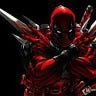# Pong Game using Pygame

## Installing pygame:

`python3 -m pip install -U pygame --user`

## Basic Implementation of Pygame:

`# import the pygame moduleimport pygame# import pygame.locals for easier# access to key coordinatesfrom pygame.locals import *# Define our square object and call super to# give it all the properties and methods of pygame.sprite.Sprite# Define the class for our square objectsclass Square(pygame.sprite.Sprite): def __init__(self):  super(Square, self).__init__()    # Define the dimension of the surface  # Here we are making squares of side 25px  self.surf = pygame.Surface((25, 25))    # Define the color of the surface using RGB color coding.  self.surf.fill((0, 200, 255))  self.rect = self.surf.get_rect()# initialize pygamepygame.init()# Define the dimensions of screen objectscreen = pygame.display.set_mode((800, 600))# instantiate all square objectssquare1 = Square()square2 = Square()square3 = Square()square4 = Square()# Variable to keep our game loop runninggameOn = True# Our game loopwhile gameOn: # for loop through the event queue for event in pygame.event.get():    # Check for KEYDOWN event  if event.type == KEYDOWN:      # If the Backspace key has been pressed set   # running to false to exit the main loop   if event.key == K_BACKSPACE:    gameOn = False      # Check for QUIT event  elif event.type == QUIT:   gameOn = False# Define where the squares will appear on the screen # Use blit to draw them on the screen surface screen.blit(square1.surf, (40, 40)) screen.blit(square2.surf, (40, 530)) screen.blit(square3.surf, (730, 40)) screen.blit(square4.surf, (730, 530))# Update the display using flip pygame.display.flip()`

## Let’s Get started:

`from time import sleepimport pygamepygame.init()WIDTH, HEIGHT =700,500WIN= pygame.display.set_mode((WIDTH, HEIGHT))pygame.display.set_caption("Pong Game")# Settinhg FPSFPS=60# Defining Color VariablesBLACK=(0,0,0)WHITE=(255,255,255)TURQUOISE=(175,238,238)PINK_ORANGE=(248, 152, 128)SADDLE_BROWN=(139,69,19)# Defining Ball VariablesMAX_VEL=6BALL_RADIUS=7# Defining font VariablesSCORE_FONT=pygame.font.SysFont("comicsans",50)WINING_SCORE=10`

## Step-2: Creating a Class for Paddles

`#Setting class for PaddlesPADDLE_WIDTH,PADDLE_HEIGHT=20,100class Paddle:    COLOR1,COLOR2=WHITE,TURQUOISE    VEL=4    def __init__(self,x,y,width,height):        self.x = self.orignal_x = x        self.y = self.orignal_y = y        self.width = width        self.height = height    def draw_paddle(self,win):        pygame.draw.rect(win,self.COLOR2,                        (self.x,self.y,self.width,self.height))    def paddle_movement(self,up=True):        if up == True:           self.y=self.y-self.VEL        else:           self.y=self.y+self.VEL    def reset_paddle(self):        self.x=self.orignal_x        self.y=self.orignal_y`

## Step-3: Creating a Class for Ball

`# Making class for Ballclass Ball:    MAX_VEL=6    COLOR=WHITE    def __init__(self,x,y,radius):        self.x =self.orignal_x = x        self.y = self.orignal_y = y        self.radius = radius        self.x_vel=MAX_VEL        self.y_vel=0    def draw_ball(self,win):        pygame.draw.circle(win,self.COLOR,(self.x,self.y),self.radius)    def move_ball(self):        self.x=self.x+self.x_vel        self.y=self.y+self.y_vel    def reset_ball(self):        self.x=self.orignal_x        self.y=self.orignal_y`

## Step-4: Handling Collision

`#defining for handle collisiondef handle_collision(ball,left_paddle,right_paddle):    # For ceiling colision    if ball.y+  ball.radius>=HEIGHT:       ball.y_vel*=-1    elif ball.y - ball.radius<=0:       ball.y_vel*=-1    #now for left_paddle and ball collision    if ball.x_vel<0:       if (ball.y>=left_paddle.y) and (ball.y<=left_paddle.y +    left_paddle.height):          if (ball.x - ball.radius) <= (left_paddle.x +  left_paddle.width):          ball.x_vel*=-1    #For ball_y_velocity on right_paddle    middle_y=left_paddle.y+ left_paddle.height//2    difference_in_y=middle_y-ball.y    reduction_factor= (left_paddle.height/2)/ball.MAX_VEL    y_vel=difference_in_y/reduction_factor    ball.y_vel=-1*y_vel    #For right_paddle and ball collision    else:      if ball.y>=right_paddle.y and ball.y<=(right_paddle.y + right_paddle.height):         if ball.x + ball.radius >= right_paddle.x:            ball.x_vel*=-1    #For ball_y_velocity on right_paddle    middle_y=left_paddle.y+ left_paddle.height//2    difference_in_y=middle_y-ball.y    reduction_factor= (left_paddle.height/2)/ball.MAX_VEL    y_vel=difference_in_y/reduction_factor    ball.y_vel=-1*y_vel`

## Step-4: Handling Key Movement

`#defining handle_movement depending on the Keysdef handle_paddle_movement(keys,left_paddle, right_paddle):    if keys[pygame.K_w] and (left_paddle.y - left_paddle.VEL>=0):       left_paddle.paddle_movement(up=True)        if keys[pygame.K_s] and (left_paddle.y + left_paddle.VEL + left_paddle.height <=HEIGHT):       left_paddle.paddle_movement(up=False)    if keys[pygame.K_UP] and (right_paddle.y - right_paddle.VEL>=0):       right_paddle.paddle_movement(up=True)    if keys[pygame.K_DOWN] and (right_paddle.y + right_paddle.VEL + right_paddle.height <=HEIGHT):       right_paddle.paddle_movement(up=False)`

## Step-4: Drawing Everything on the Window

`# Defining the person to show on Windows Finallydef draw(win,paddles,ball,left_score,right_score):    win.fill(BLACK)    #Adding score    #These two lines will give us a drawable object    left_score_text=SCORE_FONT.render(f"{left_score}",1,PINK_ORANGE)    right_score_text=SCORE_FONT.render(f"{right_score}",1,PINK_ORANGE)    #Applying the text object    win.blit(left_score_text,(WIDTH//4-left_score_text.get_width(),20))    win.blit(right_score_text,(3*WIDTH//4-right_score_text.get_width(),20))    #Adding Paddles    for paddle in paddles:        paddle.draw_paddle(win)   # Adding Dash line   for i in range(10,HEIGHT,HEIGHT//20):        if i % 2==1:           continue        pygame.draw.rect(win,PINK_ORANGE,(WIDTH//2-5,i,5,HEIGHT//20))   #Adding ball   ball.draw_ball(win)   pygame.display.update() #just like stateful widget`

## Step-6: Ouput

--

--## Rishabh

Student from B.tech 2nd Year, A proud ARTH learner, love new technologies, Curious about many thing, likes to explore places, love eating pizza and much more.